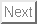: Dis45 \$B%f!<%6!<\$N\$?\$a\$N(B ROOT \$BF~Lg(B : \$B;29MJ88%(B   \$BL\

# \$B\$3\$NJ8=q\$K\$D\$\$\$F(B...

Dis45 \$B%f!<%6!<\$N\$?\$a\$N(B ROOT \$BF~Lg(B
\$B1n\$K\$b;H\$(\$k(BROOT\$B!'HV30JT(B

\$B\$3\$NJ8=q\$O(BLaTeX2HTML \$BK]Lu%W%m%0%i%`(B Version 99.1 release (March 30, 1999)

Copyright © 1993, 1994, 1995, 1996, Nikos Drakos, Computer Based Learning Unit, University of Leeds,
Copyright © 1997, 1998, 1999, Ross Moore, Mathematics Department, Macquarie University, Sydney.

\$B\$r(B\$BF|K\8l2=\$7\$?\$b\$N(B( 99.1 release + JA patch 3.0 (September 15, 1999) \$BHG(B)

\$BK]Lu\$O(B Keisuke Fujii \$B\$K\$h\$C\$F(B \$BJ?@.(B12\$BG/(B12\$B7n(B22\$BF|(B \$B\$K